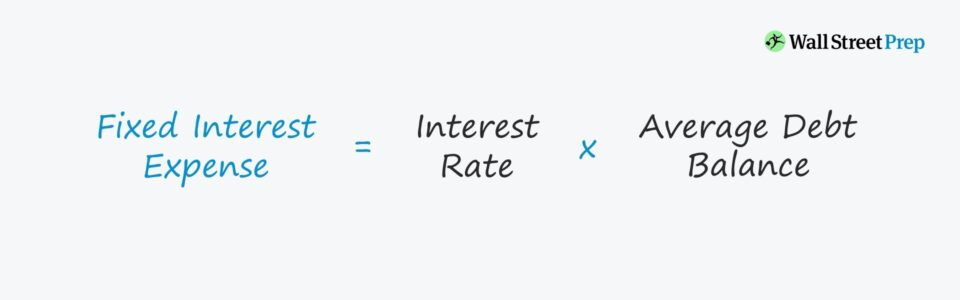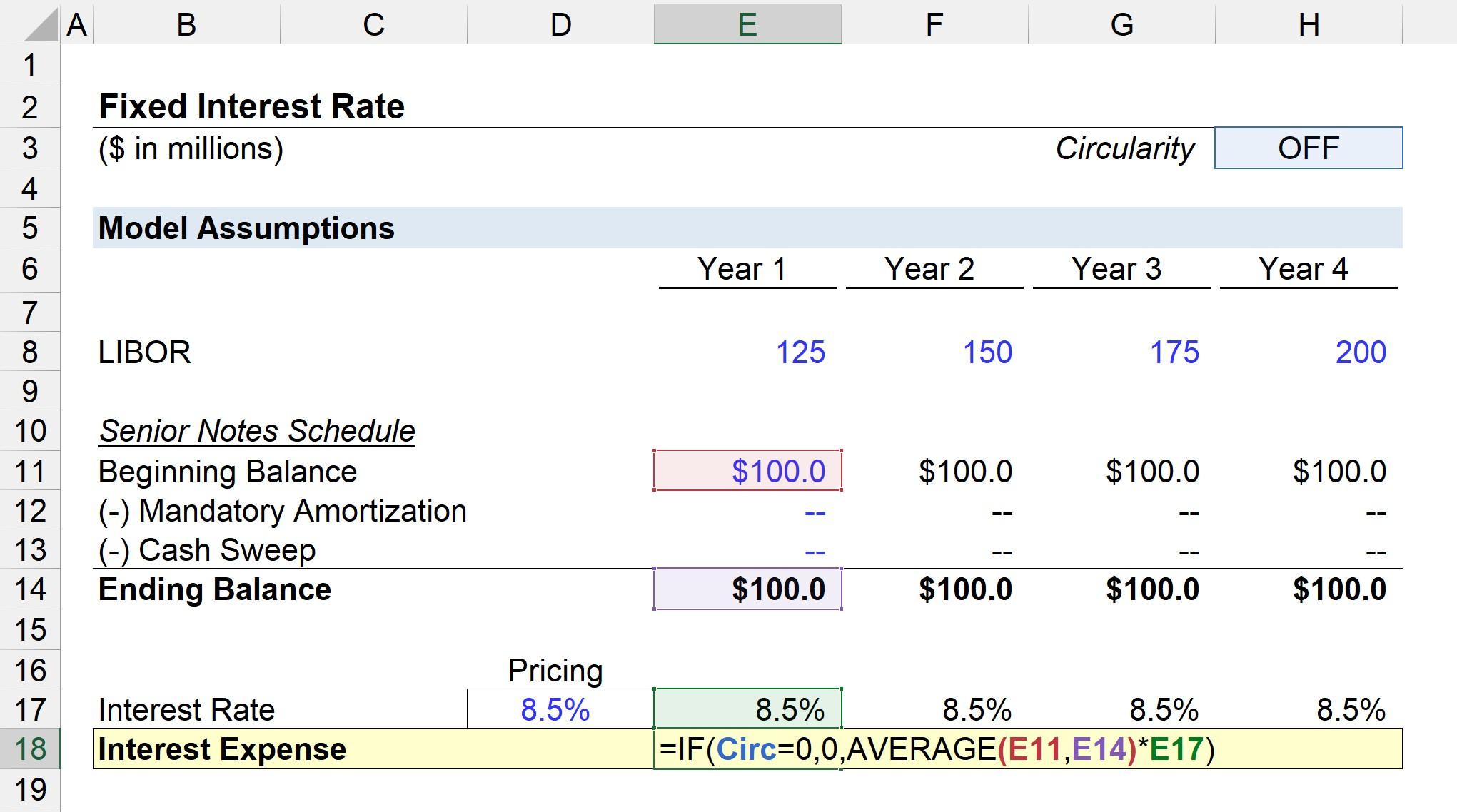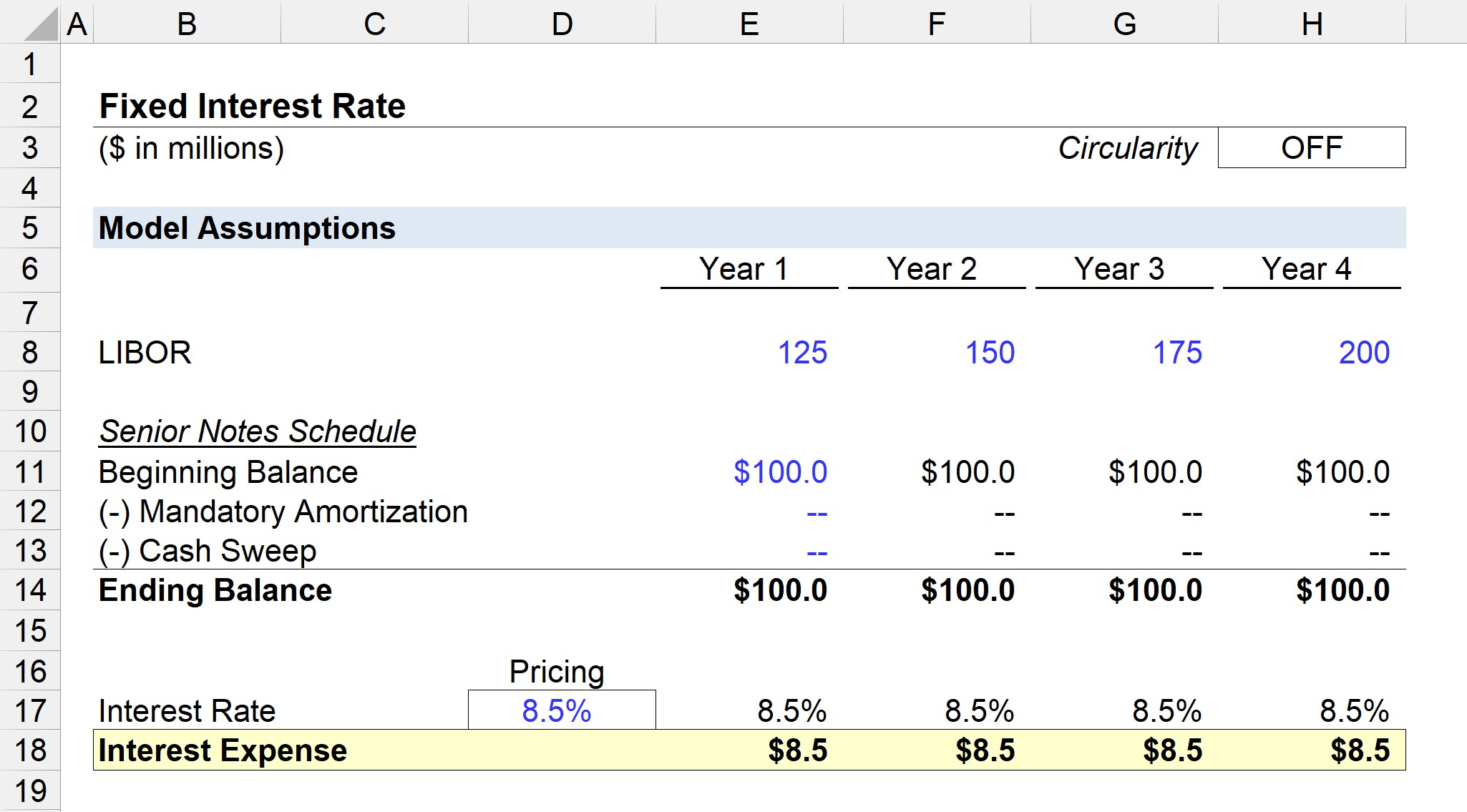Welcome to Wall Street Prep! Use code at checkout for 15% off.# Fixed Interest Rate

Guide to Understanding Fixed Interest Rates## How to Calculate Fixed Interest Rate (Step-by-Step)

If a loan or bond is priced at a fixed interest rate, the interest rate – which determines the interest expense amount due each period – is fixed and does not fluctuate over time.

In general, fixed pricing tend to be more prevalent with bonds and riskier debt instruments further down in the capital structure, rather than senior debt provided by banks.

The distinct benefit of fixed rates is the predictability in the pricing of the debt, as the borrower does not need to be concerned about changing market conditions that may impact the interest due.

The fact that the interest rate pricing structure is fixed mitigates any risk that the borrower’s interest expense payments could increase substantially.

Typically, borrowers are more likely to opt for fixed rates in lending agreements during low interest rate environments in an attempt to “lock-in” favorable borrowing terms for the long term.

## Fixed Interest Rate Formula

The formula for calculating the interest expense on a debt instrument with fixed pricing is as follows.

Interest Expense = Fixed Interest Rate (%)Average Debt Balance

## Fixed Interest Rate vs. Floating Interest Rate

Unlike fixed pricing rates, floating rates fluctuate based on the underlying benchmark rate that is tied to the pricing of the debt (e.g. LIBOR, SOFR).

The relationship between the market rate and the yield on debt priced at a floating rate is as follows.

• Declining Market Rate: If the market rate declines, the borrower benefits from the lower interest rate.
• Rising Market Rate: If the market rate rises, the lender benefits from the higher interest rate.

Floating interest rates thus can be a riskier form of debt pricing with more uncertainty because of the unpredictable changes in the underlying benchmark.

If the debt is priced on a fixed basis, the original interest rate remains the same, which eliminates any concerns from the borrower regarding how much interest will be owed.

However, the fixed pricing comes at the expense of not being able to benefit in lower interest rate environments.

For example, if the benchmark rate is lower and the lending environment becomes more favorable for borrowers, interest expense on a bond priced at a fixed rate would still stay unchanged.

## Fixed Interest Rate Calculator – Excel Template

We’ll now move to a modeling exercise, which you can access by filling out the form below.Submitting...

## Fixed Interest Rate Calculation Example

In our illustrative example, we’ll assume there is a senior note with a total outstanding balance of \$100 million.

For the sake of simplicity, there’ll be no mandatory amortization or cash sweeps (i.e. optional prepayments) across the forecast period.

For a variable interest rate, a spread is added to the market rate (e.g. LIBOR) for each corresponding year.

LIBOR Curve

• Year 1 = 125 bps
• Year 2 = 150 bps
• Year 3 = 175 bps
• Year 4 = 200 bps

But in this case, the senior notes are priced at a fixed rate of 8.5%, which is kept constant for the entirety of the forecast and multiplied by the average between the beginning and ending balance.

• Interest Rate, % = 8.5%While not relevant to our scenario because of our assumption of no mandatory amortization or cash sweep, we must add a circularity switch to offset the risk of our model malfunctioning due to the circularity created.

If the “Circ” cell is set to zero, the output is zero. But if the “Circ” cell is NOT set to zero, the output is the calculated expense using the beginning and ending balance of the company’s senior notes.

Since the balance of the senior notes does not change at all throughout the four years, the interest expense stays at \$8.5 million every year, as shown below.Step-by-Step Online Course

### Everything You Need To Master Financial Modeling

Enroll in The Premium Package: Learn Financial Statement Modeling, DCF, M&A, LBO and Comps. The same training program used at top investment banks.Inline FeedbacksLearn Financial Modeling Online

Everything you need to master financial and valuation modeling: 3-Statement Modeling, DCF, Comps, M&A and LBO.

X

The Wall Street Prep Quicklesson Series

7 Free Financial Modeling Lessons

Get instant access to video lessons taught by experienced investment bankers. Learn financial statement modeling, DCF, M&A, LBO, Comps and Excel shortcuts.